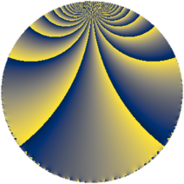# Properties

 Label 345.3.kLevel $345$ Weight $3$ Character orbit 345.k Rep. character $\chi_{345}(208,\cdot)$ Character field $\Q(\zeta_{4})$ Dimension $88$ Newform subspaces $1$ Sturm bound $144$ Trace bound $0$

# Learn more about

## Defining parameters

 Level: $$N$$ $$=$$ $$345 = 3 \cdot 5 \cdot 23$$ Weight: $$k$$ $$=$$ $$3$$ Character orbit: $$[\chi]$$ $$=$$ 345.k (of order $$4$$ and degree $$2$$) Character conductor: $$\operatorname{cond}(\chi)$$ $$=$$ $$5$$ Character field: $$\Q(i)$$ Newform subspaces: $$1$$ Sturm bound: $$144$$ Trace bound: $$0$$

## Dimensions

The following table gives the dimensions of various subspaces of $$M_{3}(345, [\chi])$$.

Total New Old
Modular forms 200 88 112
Cusp forms 184 88 96
Eisenstein series 16 0 16

## Trace form

 $$88q + 8q^{2} + 16q^{5} + 16q^{7} - 24q^{8} + O(q^{10})$$ $$88q + 8q^{2} + 16q^{5} + 16q^{7} - 24q^{8} - 8q^{10} - 48q^{12} + 56q^{13} - 48q^{15} - 256q^{16} + 24q^{17} + 24q^{18} + 112q^{20} + 48q^{21} + 48q^{22} - 168q^{25} - 304q^{26} - 312q^{28} + 144q^{30} - 88q^{31} - 128q^{32} + 144q^{33} + 168q^{35} + 528q^{36} + 320q^{38} - 416q^{40} + 264q^{41} + 96q^{42} - 128q^{43} - 72q^{45} - 184q^{47} + 192q^{48} + 120q^{50} + 32q^{52} - 288q^{53} + 216q^{55} + 288q^{56} - 48q^{57} - 328q^{58} - 272q^{61} - 128q^{62} + 48q^{63} + 32q^{65} + 144q^{66} + 592q^{67} + 656q^{68} - 152q^{70} + 552q^{71} + 72q^{72} - 192q^{73} + 144q^{75} - 464q^{76} - 88q^{77} - 336q^{78} + 224q^{80} - 792q^{81} + 568q^{82} + 288q^{83} + 296q^{85} - 352q^{86} - 144q^{87} - 312q^{88} + 192q^{90} - 528q^{91} - 896q^{95} - 632q^{97} - 192q^{98} + O(q^{100})$$

## Decomposition of $$S_{3}^{\mathrm{new}}(345, [\chi])$$ into newform subspaces

Label Dim. $$A$$ Field CM Traces $q$-expansion
$$a_2$$ $$a_3$$ $$a_5$$ $$a_7$$
345.3.k.a $$88$$ $$9.401$$ None $$8$$ $$0$$ $$16$$ $$16$$

## Decomposition of $$S_{3}^{\mathrm{old}}(345, [\chi])$$ into lower level spaces

$$S_{3}^{\mathrm{old}}(345, [\chi]) \cong$$ $$S_{3}^{\mathrm{new}}(15, [\chi])$$$$^{\oplus 2}$$$$\oplus$$$$S_{3}^{\mathrm{new}}(115, [\chi])$$$$^{\oplus 2}$$